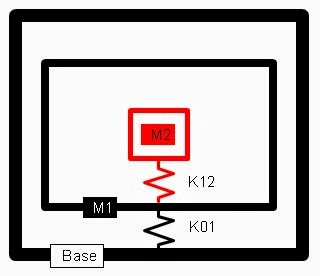## Wednesday, January 22, 2014

### Vibration Isolation in a Random Vibration Environment

Attached is an MS Excel Macro of a 2 degree-of-freedom vibration simulation model that calculates the response to a random vibration input - PSD (power spectral density).2-DOF Random Vibration Dashboard.
Vibration isolation strategy for tonal systems is generally straight forward where one can isolate by using soft isolators that put the forcing frequencies above the isolation frequency. Or tune between forcing frequencies. Sway space, damping, isolator fatigue and other considerations are needed as well.

For random vibration input the strategy is not so straight forward.  In theory, all frequencies between the limits of the PSD are present.  Any system natural frequency within this range would be set into resonance.  Sway space, damping and other considerations are still important.Typical PSD Curve.
The simulation model of the macro uses 2 masses with the random vibration input at the base.  It could simulate the frame of a car that has an electrical containment box attached to it that in turn has a circuit board mounted inside the box.  The circuit board is depicted as body (mass) 2, the box depicted as body (mass) 1 and the car body the rigid body that randomly vibrates the box from road irregularities, drive-train, and other sources..2 DOF Vibration Model.
The simulation model has the following features:
1. M1, M2, K01 and K12 are input by the user.
2. Modal damping ratio can be input.
3. A PSD curve can be input.
4. A user defined gravitational constant is input.
5. Any consistent set of dimensional units can be used.

The simulation can calculate:
1. PSD Grms value; the 2 natural frequencies and mode shapes.
2. Mode shapes can be animated.
3. RMS response G's and relative displacement values.
4. A pseudo time series can be determined from the PSD.
5. A dynamic transient analysis of the  time series can be done and response values determined.
6. The transient response can be animated.Random Vibration Response.

For a typical example the following was simulated: a 5 lb circuit board mounted inside a 50 lb box which is mounted to a car frame that has a uniform 5-500 Hz 0.5 G2/Hz random vibration input. Low modal damping of 2% was also assumed. Two scenarios were evaluated.

First, it was assumed the circuit board was on mounts and/or isolators that gave it a natural frequency of 25 Hz.  The box was then mounted to the car frame by a progressively softer attachment.  Starting virtually hard mounted and then softened to a a frequency near the 25 Hz.  The results are shown below.  The box acceleration response is initially high because it's primary mode (box-to-car frame mode) is within the 500 Hz PSD range.  As its mounting gets softer that mode drops in frequency and the response drops.  As that frequency gets closer to the circuit board mode frequency they start to dynamically couple and more response is felt by the circuit board.  As the frequency ratio (2nd mode-to-1st mode) approaches 2 from the right side of the chart the circuit response starts to increase dramatically.Body 2 at 25 Hz and Body 1 Mounted with Varying Stiffnesses.
The second scenario was to assume the box-to-car frame natural frequency is 25 Hz and the circuit board mounted progressively softer from starting with a "hard" mount case. The results are below.

The trends are similar to the first scenario except that the box response starts out relatively low because it's already at a low natural frequency and the circuit board is isolated by the box from the car.  As the circuit board mounting becomes softer the acceleration response of both the box and circuit board increase.  At a frequency ratio of 2 they are feeling large responses from their mode coupling.  In this case the mode 2 is predominately the circuit board mode.  Whereas in the first scenario the box was predominately mode 2.

The take ways from this simple study are: 1) For 2 DOF system keeping the 2 modes separated by a frequency ratio of at least 2 (an octave) or more would be desirable from an acceleration response prospective.  A frequency ratio greater than 4 appears to offer diminishing returns. 2) Isolating the box is preferred over isolating the circuit board assuming no other considerations make this undesirable like available sway space for the box which increases as the isolation frequency drops.

The attached spreadsheet summarizes the details shown in the two preceding charts.

Of course, other factors need to be evaluated.  For example, this simple case used a uniform PSD input.  Most PSD's decrease at the higher frequencies.  Additional damping can be used, especially if elastomer isolators are used.

Note:  The MS Excel Macro has been code signed with an independent code signing certificate to allow higher security settings.  The macro has a time limit of 1/1/2015.  Updated versions will be accessible at www.XL4Sim.com.

1.